ARTS  2.4.0(git:4fb77825)
Zeeman Namespace Reference

Implements Zeeman modeling. More...

## Classes

struct  AllPolarizationVectors
PolarizationVector for each Polarization. More...

struct  Derived
Contains derived values useful for Zeeman calculations. More...

class  Model
Main Zeeman Model. More...

class  PolarizationVector
Polarization vector for Zeeman Propagation Matrix. More...

struct  SplittingData
Main storage for Zeeman splitting coefficients. More...

## Enumerations

enum  Polarization { Polarization::SigmaMinus, Polarization::Pi, Polarization::SigmaPlus }
Zeeman polarization selection. More...

## Functions

constexpr Index dM (Polarization type) noexcept
Gives the change of M given a polarization type. More...

constexpr Rational start (Rational Ju, Rational Jl, Polarization type) noexcept
Gives the lowest M for a polarization type of this transition. More...

constexpr Rational end (Rational Ju, Rational Jl, Polarization type) noexcept
Gives the largest M for a polarization type of this transition. More...

constexpr Index nelem (Rational Ju, Rational Jl, Polarization type) noexcept
Gives the number of elements of the polarization type of this transition. More...

constexpr Rational Mu (Rational Ju, Rational Jl, Polarization type, Index n) noexcept
Gives the upper state M value at an index. More...

constexpr Rational Ml (Rational Ju, Rational Jl, Polarization type, Index n) noexcept
Gives the lower state M value at an index. More...

constexpr Numeric PolarizationFactor (Polarization type) noexcept
The renormalization factor of a polarization type. More...

constexpr bool GoodHundData (const QuantumNumbers &qns) noexcept
Checks if the quantum numbers are good for this transition. More...

constexpr Numeric SimpleGCaseB (Rational N, Rational J, Rational Lambda, Rational S, Numeric GS, Numeric GL) noexcept
Computes the Zeeman splitting coefficient. More...

constexpr Numeric SimpleGCaseA (Rational Omega, Rational J, Rational Lambda, Rational Sigma, Numeric GS, Numeric GL) noexcept
Computes the Zeeman splitting coefficient. More...

constexpr Numeric SimpleG (const QuantumNumbers &qns, const Numeric &GS, const Numeric &GL) noexcept
Computes the Zeeman splitting coefficient. More...

Model GetSimpleModel (const QuantumIdentifier &qid) noexcept
Returns a simple Zeeman model. More...

Model GetAdvancedModel (const QuantumIdentifier &qid) noexcept
Returns an advanced Zeeman model. More...

std::ostream & operator<< (std::ostream &os, const Model &m)

std::istream & operator>> (std::istream &is, Model &m)

std::ostream & operator<< (bofstream &bof, const Model &m)

std::istream & operator>> (bifstream &bif, Model &m)

AllPolarizationVectors AllPolarization (Numeric theta, Numeric eta) noexcept
Computes the polarization of each polarization type. More...

AllPolarizationVectors AllPolarization_dtheta (Numeric theta, const Numeric eta) noexcept
The derivative of AllPolarization wrt theta. More...

AllPolarizationVectors AllPolarization_deta (Numeric theta, Numeric eta) noexcept
The derivative of AllPolarization wrt eta. More...

const PolarizationVectorSelectPolarization (const AllPolarizationVectors &data, Polarization type) noexcept
Selects the polarization vector depending on polarization type. More...

Derived FromGrids (Numeric u, Numeric v, Numeric w, Numeric z, Numeric a) noexcept
Computes the derived plane from ARTS grids. More...

constexpr Derived FromPreDerived (Numeric H, Numeric theta, Numeric eta) noexcept
Sets Derived from predefined Derived parameters. More...

## Detailed Description

Implements Zeeman modeling.

## ◆ Polarization

 enum Zeeman::Polarization
strong

Zeeman polarization selection.

Enumerator
SigmaMinus
Pi
SigmaPlus

Definition at line 43 of file zeemandata.h.

## ◆ AllPolarization()

 AllPolarizationVectors Zeeman::AllPolarization ( Numeric theta, Numeric eta )
inlinenoexcept

Computes the polarization of each polarization type.

Parameters
 [in] theta The angle along the magnetic field [in] eta The angle counter-clockwise in the magnetic field plane
Returns
The polarization vectors of all Zeeman polarization

Definition at line 578 of file zeemandata.h.

Referenced by zeeman_on_the_fly().

## ◆ AllPolarization_deta()

 AllPolarizationVectors Zeeman::AllPolarization_deta ( Numeric theta, Numeric eta )
inlinenoexcept

The derivative of AllPolarization wrt eta.

Parameters
 [in] theta The angle along the magnetic field [in] eta The angle counter-clockwise in the magnetic field plane
Returns
The derivative of AllPolarization wrt eta

Definition at line 625 of file zeemandata.h.

Referenced by zeeman_on_the_fly().

## ◆ AllPolarization_dtheta()

 AllPolarizationVectors Zeeman::AllPolarization_dtheta ( Numeric theta, const Numeric eta )
inlinenoexcept

The derivative of AllPolarization wrt theta.

Parameters
 [in] theta The angle along the magnetic field [in] eta The angle counter-clockwise in the magnetic field plane
Returns
The derivative of AllPolarization wrt theta

Definition at line 601 of file zeemandata.h.

Referenced by zeeman_on_the_fly().

## ◆ dM()

 constexpr Index Zeeman::dM ( Polarization type )
constexprnoexcept

Gives the change of M given a polarization type.

Parameters
 [in] type The polarization type
Returns
The change in M

Definition at line 51 of file zeemandata.h.

References max, and SigmaMinus.

Referenced by Ml(), and Zeeman::Model::Strength().

## ◆ end()

 constexpr Rational Zeeman::end ( Rational Ju, Rational Jl, Polarization type )
constexprnoexcept

Gives the largest M for a polarization type of this transition.

Since the polarization determines the change in M, this function gives the last M of interest in the range of M possible for a given transition

The user has to ensure that Ju and Jl is a valid transition

Parameters
 [in] Ju J of the upper state [in] Jl J of the upper state [in] type The polarization type
Returns
The largest M value

Definition at line 108 of file zeemandata.h.

References max, min, and SigmaMinus.

## ◆ FromGrids()

 Zeeman::Derived Zeeman::FromGrids ( Numeric u, Numeric v, Numeric w, Numeric z, Numeric a )
noexcept

Computes the derived plane from ARTS grids.

When done and if everything is well-defined: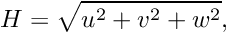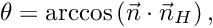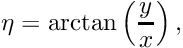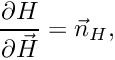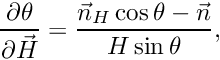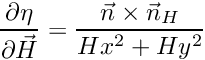With these helpers (some defined, others not):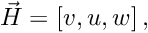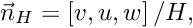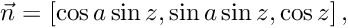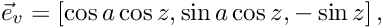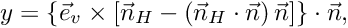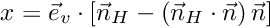Note that all other values are zero if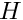is zero, that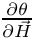is zero if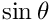is zero, thatis zero if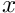and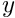are zero, and that the atan2(y, x) function is used for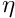.

Parameters
 [in] u Magnetic field u-parameter [in] v Magnetic field b-parameter [in] w Magnetic field w-parameter [in] z Zenith angle [in] a Azimuth angle
Returns
The derived plane

Definition at line 236 of file zeemandata.cc.

Referenced by zeeman_on_the_fly().

## ◆ FromPreDerived()

 constexpr Derived Zeeman::FromPreDerived ( Numeric H, Numeric theta, Numeric eta )
constexprnoexcept

Sets Derived from predefined Derived parameters.

Parameters
 [in] H Derived magnetic field strength [in] theta Derived magnetic field theta angle [in] eta Derived magnetic field eta angle
Returns
The pre-derived plane

Definition at line 712 of file zeemandata.h.

Referenced by FromGrids(), and zeeman_on_the_fly().

 Zeeman::Model Zeeman::GetAdvancedModel ( const QuantumIdentifier & qid )
noexcept

Will look at available Quantum numbers and use best approximation for the model to use. If no good approximation is available, it returns Model({0, 0}).

Parameters
 [in] qid Transition type quantum id
Returns
Zeeman model

Definition at line 105 of file zeemandata.cc.

References Isotopologue, and v1.

## ◆ GetSimpleModel()

 Zeeman::Model Zeeman::GetSimpleModel ( const QuantumIdentifier & qid )
noexcept

Returns a simple Zeeman model.

Will use the simple Hund case provided by input. Throws if the input is bad

Parameters
 [in] qid Transition type quantum id
Returns
Zeeman model

Definition at line 33 of file zeemandata.cc.

References get_lande_lambda_constant(), get_lande_spin_constant(), gu, and SimpleG().

Referenced by Zeeman::Model::Model(), and test_zeeman().

## ◆ GoodHundData()

 constexpr bool Zeeman::GoodHundData ( const QuantumNumbers & qns )
constexprnoexcept

Checks if the quantum numbers are good for this transition.

Given some Hund state, various quantum numbers must be defined to allow the Zeeman calculations to work

Parameters
 [in] qns Quantum numbers of a level
Returns
If the numbers can be used to compute simple Zeeman effect

Definition at line 212 of file zeemandata.h.

References CaseA, J, and N.

Referenced by SimpleG().

## ◆ Ml()

 constexpr Rational Zeeman::Ml ( Rational Ju, Rational Jl, Polarization type, Index n )
constexprnoexcept

Gives the lower state M value at an index.

The user has to ensure that Ju and Jl is a valid transition

The user has to ensure n is less than the number of elements

Parameters
 [in] Ju J of the upper state [in] Jl J of the upper state [in] type The polarization type [in] n The position
Returns
The lower state M

Definition at line 173 of file zeemandata.h.

References dM(), and Mu().

Referenced by Zeeman::Model::Strength().

## ◆ Mu()

 constexpr Rational Zeeman::Mu ( Rational Ju, Rational Jl, Polarization type, Index n )
constexprnoexcept

Gives the upper state M value at an index.

The user has to ensure that Ju and Jl is a valid transition

The user has to ensure n is less than the number of elements

Parameters
 [in] Ju J of the upper state [in] Jl J of the upper state [in] type The polarization type [in] n The position
Returns
The upper state M

Definition at line 152 of file zeemandata.h.

References start().

Referenced by Ml(), and Zeeman::Model::Strength().

## ◆ nelem()

 constexpr Index Zeeman::nelem ( Rational Ju, Rational Jl, Polarization type )
constexprnoexcept

Gives the number of elements of the polarization type of this transition.

The user has to ensure that Ju and Jl is a valid transition

Parameters
 [in] Ju J of the upper state [in] Jl J of the upper state [in] type The polarization type
Returns
The number of elements

Definition at line 135 of file zeemandata.h.

References end(), and start().

## ◆ operator<<() [1/2]

 std::ostream& Zeeman::operator<< ( bofstream & bof, const Model & m )
inline

Definition at line 493 of file zeemandata.h.

## ◆ operator<<() [2/2]

 std::ostream& Zeeman::operator<< ( std::ostream & os, const Model & m )
inline

Definition at line 483 of file zeemandata.h.

## ◆ operator>>() [1/2]

 std::istream& Zeeman::operator>> ( bifstream & bif, Model & m )
inline

Definition at line 498 of file zeemandata.h.

## ◆ operator>>() [2/2]

 std::istream& Zeeman::operator>> ( std::istream & is, Model & m )
inline

Definition at line 488 of file zeemandata.h.

## ◆ PolarizationFactor()

 constexpr Numeric Zeeman::PolarizationFactor ( Polarization type )
constexprnoexcept

The renormalization factor of a polarization type.

The polarization comes from some geometry. This function returns the factor we need to compute that geometry and to turn it into something that normalizes every possible M for this type into some strength that sums to unity

Parameters
 [in] type The polarization type
Returns
Rescale factor

Definition at line 191 of file zeemandata.h.

References max, and SigmaMinus.

Referenced by Zeeman::Model::Strength().

## ◆ SelectPolarization()

 const PolarizationVector& Zeeman::SelectPolarization ( const AllPolarizationVectors & data, Polarization type )
inlinenoexcept

Selects the polarization vector depending on polarization type.

Parameters
 [in] data The pre-computed polarization vectors [in] type The type of polarization to select

Definition at line 646 of file zeemandata.h.

References data, and SigmaMinus.

Referenced by zeeman_on_the_fly().

## ◆ SimpleG()

 constexpr Numeric Zeeman::SimpleG ( const QuantumNumbers & qns, const Numeric & GS, const Numeric & GL )
constexprnoexcept

Computes the Zeeman splitting coefficient.

The level should be Hund case a or b type and all the quantum numbers have to be defined

Parameters
 [in] qns Quantum numbers of a level [in] GS The spin Landé coefficient of the molecule [in] GS The Landé coefficient of the molecule
Returns
If the numbers can be used to compute simple Zeeman effect

Definition at line 315 of file zeemandata.h.

References CaseA, GoodHundData(), J, N, SimpleGCaseA(), and SimpleGCaseB().

Referenced by GetSimpleModel().

## ◆ SimpleGCaseA()

 constexpr Numeric Zeeman::SimpleGCaseA ( Rational Omega, Rational J, Rational Lambda, Rational Sigma, Numeric GS, Numeric GL )
constexprnoexcept

Computes the Zeeman splitting coefficient.

The level should be Hund case a type and all the values have to be defined

Parameters
 [in] Omega The Omega quantum number of the level [in] J The J quantum number of the level [in] Lambda The Lambda quantum number of the level [in] Sigma The Sigma quantum number of the level [in] GS The spin Landé coefficient of the molecule [in] GL The Landé coefficient of the molecule
Returns
Zeeman splitting coefficient of the level

Definition at line 286 of file zeemandata.h.

Referenced by SimpleG().

## ◆ SimpleGCaseB()

 constexpr Numeric Zeeman::SimpleGCaseB ( Rational N, Rational J, Rational Lambda, Rational S, Numeric GS, Numeric GL )
constexprnoexcept

Computes the Zeeman splitting coefficient.

The level should be Hund case b type and all the values have to be defined

Parameters
 [in] N The N quantum number of the level [in] J The J quantum number of the level [in] Lambda The Lambda quantum number of the level [in] S The S quantum number of the level [in] GS The spin Landé coefficient of the molecule [in] GL The Landé coefficient of the molecule
Returns
Zeeman splitting coefficient of the level

Definition at line 249 of file zeemandata.h.

References N.

Referenced by SimpleG().

## ◆ start()

 constexpr Rational Zeeman::start ( Rational Ju, Rational Jl, Polarization type )
constexprnoexcept

Gives the lowest M for a polarization type of this transition.

Since the polarization determines the change in M, this function gives the first M of interest in the range of M possible for a given transition

The user has to ensure that Ju and Jl is a valid transition

Parameters
 [in] Ju J of the upper state [in] Jl J of the upper state [in] type The polarization type
Returns
The lowest M value

Definition at line 77 of file zeemandata.h.

References max, min, and SigmaMinus.